## CONE

 Draws a cone-shaped object on an image.

 USAGE: cone [-s size] [-r radii] [-c center] [-a apex] [-e ecolors] [-t tcolors] [-b bcolor] [-m mode] [infile] outfile USAGE: cone [-help or -h] -s .... size ....... size of background image to create if no input image .................... is provided; The size=WIDTHxHEIGHT; Default=300x300 -r .... radii ...... x,y radii for the ellipse; comma separated list of .................... positive integers; The default="50,50" (circle of .................... radius=50). -c .... center ..... center coordinates for the ellipse part of the cone; .................... comma separate list of non-negative integers; .................... center="xc,yc"; default="150,100". -a .... apex ....... apex coordinates for the triangle tip of the cone; .................... comma separate list of non-negative integers; .................... center="x,y"; default="150,250". -e .... ecolors .... comma separate list of ellipse fill and stroke colors .................... and strokewidth; default="white,black,1"; -t .... tcolors .... comma separate list of triangle fill and stroke colors .................... and strokewidth; default="tan,black,1". -b .... bcolor ..... background color for image if no input image is provided; .................... default="lightblue". -m .... mode ....... mode of drawing order; choices are 1 or 2. A value of 1 .................... draws the triangle over the ellipse; A value of 2 draws .................... the ellipse over the triangle; default=1. If infile is provided, then size and bcolor will be ignored. PURPOSE: To draw a cone-shaped object on an image. DESCRIPTION: CONE draws a cone-shaped object on an image. The cone is composed of two parts an ellipse (or circle) and a triangle. One can specify different colors for each along with stroke colors and strokewidths. ARGUMENTS: -s size ... SIZE of background image to create if no input image is provided. The size=WIDTHxHEIGHT; If only one value is provided, the second will be set equal to the first. Default="300x300". -r radii ... RADII are the x,y radii for the ellipse. Radii are specified as a comma separated list of two positive integers. If only one value is provided, the second will be set equal to the first. The default="50,50" (i.e., circle of radius=50). -c center ... CENTER coordinates for the ellipse part of the cone. Center is a comma separate list of two non-negative integers. If only one value is provided, the second will be set equal to the first. The default="150,100". -a apex ... APEX coordinates for the triangle tip of the cone. Apex is a comma separate list of two non-negative integers. If only one value is provided, the second will be set equal to the first. The default="150,250". -e ecolors ... ECOLORS is a comma separate list of thre values, namely, the ellipse fill and stroke colors and strokewidth. All 3 values must be provided. The default="white,black,1"; -t tcolors ... TCOLORS is a comma separate list of thre values, namely, the triangle fill and stroke colors and strokewidth. All 3 values must be provided. The default="tan,black,1"; -b bcolor ... BCOLOR is the background color for image if no input image is provided. The default="lightblue". -m mode ... MODE of drawing order. The choices are 1 or 2. A value of 1 draws the triangle over the ellipse. A value of 2 draws the ellipse over the triangle. The default=1. CAVEAT: No guarantee that this script will work on all platforms, nor that trapping of inconsistent parameters is complete and foolproof. Use At Your Own Risk.

### EXAMPLES

 Arguments: -m 1 -r "50,50" -a "150,250" -c "150,100" -t "tan,black,1" -e "white,black,1" -b lightblue Arguments: -m 2 -r "50,50" -a "150,250" -c "150,100" -t "tan,black,1" -e "white,black,1" -b lightblue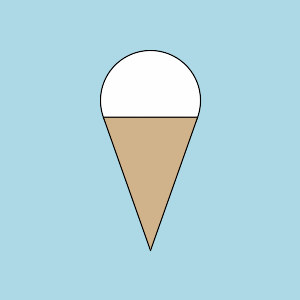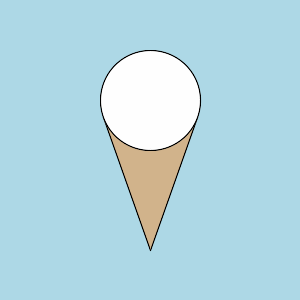Arguments: -m 1 -r "70,70" -a "150,250" -c "150,100" -t "tan,black,1" -e "white,black,1" -b lightblue Arguments: -m 2 -r "70,70" -a "150,250" -c "150,100" -t "tan,black,1" -e "white,black,1" -b lightblue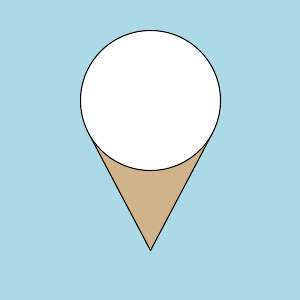Arguments: -m 1 -r "70,70" -a "250,50" -c "100,200" -t "tan,black,1" -e "white,black,1" -b lightblue Arguments: -m 2 -r "70,70" -a "250,50" -c "100,200" -t "tan,black,1" -e "white,black,1" -b lightblueArguments: -m 1 -r "50,30" -a "150,250" -c "150,100" -t "tan,black,1" -e "white,black,1" -b lightblue Arguments: -m 2 -r "50,30" -a "150,250" -c "150,100" -t "tan,black,1" -e "white,black,1" -b lightblue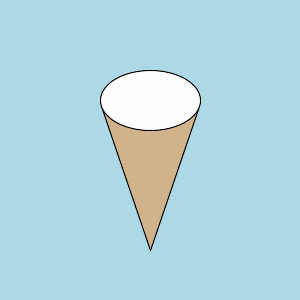Arguments: -m 1 -r "50,80" -a "150,250" -c "150,100" -t "tan,black,1" -e "white,black,1" -b lightblue Arguments: -m 2 -r "50,80" -a "150,250" -c "150,100" -t "tan,black,1" -e "white,black,1" -b lightblueArguments: -m 1 -r "50,30" -a "250,50" -c "100,200" -t "tan,black,1" -e "white,black,1" -b lightblue Arguments: -m 2 -r "50,30" -a "250,50" -c "100,200" -t "tan,black,1" -e "white,black,1" -b lightblue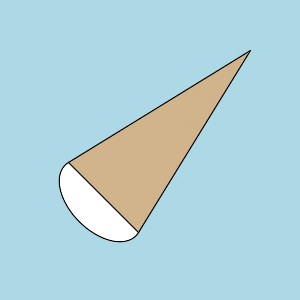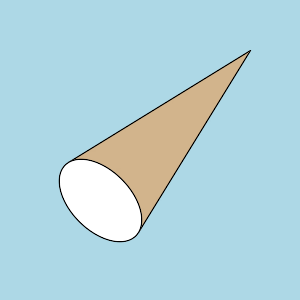Arguments: -m 1 -r "50,80" -a "250,50" -c "100,200" -t "tan,black,1" -e "white,black,1" -b lightblue Arguments: -m 2 -r "50,80" -a "250,50" -c "100,200" -t "tan,black,1" -e "white,black,1" -b lightblueWhat the script does is as follows: Calculates distance from apex to center point of ellipse Calculates rotation angle of line between apex and center point Computes tangent intersections from apex to ellipse assuming the ellipse is centered at origin and apex is above it Rotates and translates the tangent points Draws triangle from apex points and two tangent intersections Draws rotated ellipse at specified center with given radii This is equivalent to the following IM commands for mode=1: apex=`echo "\$apex" | sed 's/ //g'` x1=`echo "\$apex" | cut -d, -f 1` y1=`echo "\$apex" | cut -d, -f 2` center=`echo "\$center" | sed 's/ //g'` xc=`echo "\$center" | cut -d, -f 1` yc=`echo "\$center" | cut -d, -f 2` radii=`echo "\$radii" | sed 's/ //g'` a=`echo "\$radii" | cut -d, -f 1` b=`echo "\$radii" | cut -d, -f 2` tcolors=`echo "\$tcolors" | sed 's/ //g'` tcolor=`echo "\$tcolors" | cut -d, -f 1` tstroke=`echo "\$tcolors" | cut -d, -f 2` twidth=`echo "\$tcolors" | cut -d, -f 3` ecolors=`echo "\$ecolors" | sed 's/ //g'` enum=`echo "\$ecolors" | tr "," " " | wc -w` ecolor=`echo "\$ecolors" | cut -d, -f 1` estroke=`echo "\$ecolors" | cut -d, -f 2` ewidth=`echo "\$ecolors" | cut -d, -f 3` x2=`convert xc: -format "%[fx:\$xc-\$a]" info:` y2=\$yc x3=`convert xc: -format "%[fx:\$xc+\$a]" info:` y3=\$yc len=`convert xc: -format "%[fx:hypot((\$y1-\$yc),(\$x1-\$xc))]" info:` angle=`convert xc: -format "%[fx:(180/pi)*atan2((\$x1-\$xc),(\$yc-\$y1))]" info:` x4=0 y4=-\$len x5=\$x2 y5=0 x6=\$x3 y6=0 A=`convert xc: -format "%[fx:\$a*\$a*\$y4*\$y4 + \$b*\$b*\$x4*\$x4]" info:` B=`convert xc: -format "%[fx:-2*\$a*\$a*\$b*\$b*\$x4]" info:` C=`convert xc: -format "%[fx:\$a*\$a*\$a*\$a*(\$b*\$b-\$y4*\$y4)]" info:` x7=`convert xc: -format "%[fx:(-\$B-sqrt(\$B*\$B-4*\$A*\$C))/(2*\$A)]" info:` x8=`convert xc: -format "%[fx:(-\$B+sqrt(\$B*\$B-4*\$A*\$C))/(2*\$A)]" info:` y7=`convert xc: -format "%[fx:(\$a*\$a*\$b*\$b-\$b*\$b*\$x4*\$x7)/(\$a*\$a*\$y4)]" info:` y8=`convert xc: -format "%[fx:(\$a*\$a*\$b*\$b-\$b*\$b*\$x4*\$x8)/(\$a*\$a*\$y4)]" info:` x9=`convert xc: -format "%[fx:(\$x7)cos(pi*\$angle/180)-(\$y7+\$len)sin(pi*\$angle/180)+\$x1]" info:` y9=`convert xc: -format "%[fx:(\$x7)sin(pi*\$angle/180)+(\$y7+\$len)cos(pi*\$angle/180)+\$y1]" info:` x10=`convert xc: -format "%[fx:(\$x8)cos(pi*\$angle/180)-(\$y8+\$len)sin(pi*\$angle/180)+\$x1]" info:` y10=`convert xc: -format "%[fx:(\$x8)sin(pi*\$angle/180)+(\$y8+\$len)cos(pi*\$angle/180)+\$y1]" info:` convert \$input \ -fill \$ecolor -stroke \$estroke -strokewidth \$ewidth \ -draw "translate \$xc,\$yc rotate \$angle ellipse 0,0 \$a,\$b 0,360" \ -fill \$tcolor -stroke \$tstroke -strokewidth \$twidth \ -draw "polygon \$x1,\$y1 \$x9,\$y9 \$x10,\$y10" \ \$outfile